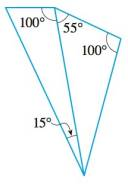Chapter A, Problem 8E### Algebra and Trigonometry (MindTap ...

4th Edition
James Stewart + 2 others
ISBN: 9781305071742

#### Solutions

Chapter
Section### Algebra and Trigonometry (MindTap ...

4th Edition
James Stewart + 2 others
ISBN: 9781305071742
Textbook Problem

# 5-8 Similar Triangles? Determine whether the pair of triangles is similar.To determine

Whether the pair of triangles is similar or not.

Explanation

Given:

The given pair of triangle is,

Figure (1)

Approach:

Two triangles are similar if their vertices can be matched up so that corresponding angles are congruent. In this case corresponding sides are proportional.

Calculation:

Here, the unknown angle in the first triangle is,

180°15°

### Still sussing out bartleby?

Check out a sample textbook solution.

See a sample solution

#### The Solution to Your Study Problems

Bartleby provides explanations to thousands of textbook problems written by our experts, many with advanced degrees!

Get Started